# Draw and Describe The Frequency – Domain Representation or Frequency Spectrum of AM Waves

## Frequency Spectrum of AM Waves

### Mathematical Expression and Waveforms

Let x(t) is the modulating signal and the carrier signal is given by the expression:

C(t) = A cos ωct         ……………….(1)

Then the equation for the AM will be

s(t) = x(t) cos ωct + A cos ωct   ……….(2)

This equation describes the AM wave in time-domain. However, if we want to know the frequency description or frequencies present in AM wave, we will have to find its spectrum or frequency-domain representation.

For this purpose, first we have to take the Fourier transform of AM wave.

Let  S(ω) denote the Fourier transform of s(t),

C(ω) denotes the Fourier transform of c(t)

X(ω) denoted the Fourier transform of x(t).

Let the modulating signal x(t) and its Fourier transform X(ω) be shown in figure  1 (a) .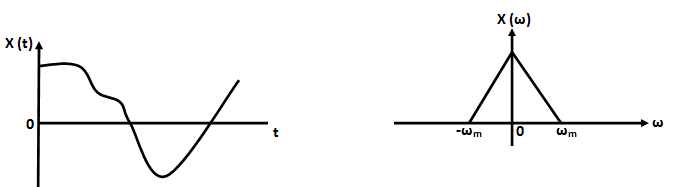Fig 1 (a)

Let the modulating signal or message signal x(t) be band-limited to the interval :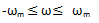This means that the modulating signal does not have any frequency component outside the interval (-ωm ,  ωm).

It may be noted at this point that in figure 1 (a) the modulating signal frequency ranges extend from -ωm to  ωm i.e. it includes negative frequencies also from -ωm to 0 . Practically there is no meaning of negative frequency. In fact, the negative frequency is used for mathematical convenience only.

Hence we can say that the modulating signal contains frequencies from 0 to ωm or simply the bandwidth of modulating signal is ωm . We know that the Fourier transform of a cosine signal cos ωct   consists of two impulse at ωc and – ωc as :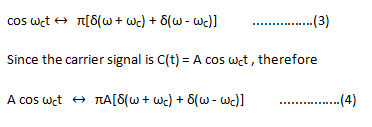Figure 1 (b) shows the carrier signal A cos ωct   and its Fourier transform.Now, the Am wave is given as :

s(t) = x(t) cos ct   + A cos ωct   ………(5)

To find the spectrum of AM wave, we take its Fourier transform.

The fourier transform of s(t) may be found by considering the two factors x(t) cos ωct and A cos ωct      separately as follows:

To find the Fourier transform of x(t) cos ωct , we first note the frequency-shifting theorem of Fourier transform as  :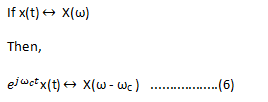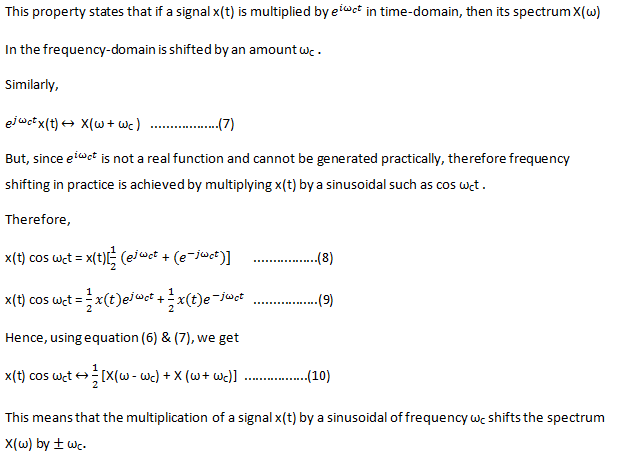The Fourier transform of the second factor A cos ωct will be as in equation (4)Therefore, the Fourier transform of AM wave given by equation (2) will be the sum of equations (10) and (11).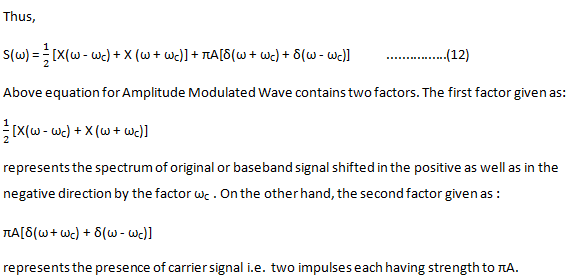Thus the spectrum of modulated signal contains shifted spectrum of modulating signal and the spectrum of carrier signal as shown in figure 1 (c) .Fig 1(c)

### Important Points

From figure 1 (c) following points may be observed:

1. For positive frequencies, a portion of the spectrum of AM wave is lying above the carrier frequency ωc . This band of frequency which is lying above the carrier frequency ωc is known as the Upper Sideband (USB) whereas the symmetrical portion below carrier frequency ωc is known as the Lower Sideband (LSB) . For negative frequencies, the upper sideband (USB) is represented by the portion of the spectrum below -ωc and the lower sideband by the symmetrical portion above -ωc . Two sidebands have been shown in figure 1 (c).
2. We generally keep ωc > ωm which ensures that the two sidebands do not overlap each other.
3. At this point, it may be noted that the negative frequencies appeared in spectrum analysis is due to the exponential representation of Fourier Transform. These negative frequencies are used for mathematical convenience. For a real function, these negative frequencies have no meaning. For general purpose, it is sufficient to consider only the positive frequency region while treating the negative frequency region as a replica of the positive frequencies. This means that to calculate the BW of a signal, we consider only positive side.
4. From figure, it is obvious that for positive side, the highest frequency component present in the spectrum of AM wave is ωc + ωm and the lowest frequency component is ωc – ωm. Infact, the difference between these two extreme frequencies is equal to the bandwidth of the AM wave.

Therefore, Bandwidth

B = (ωc + ωm ) – ( ωc – ωm )

B = 2ωm

Thus, it is clear that the bandwidth of the amplitude modulated wave is twice the highest frequency present in the baseband or modulating signal.